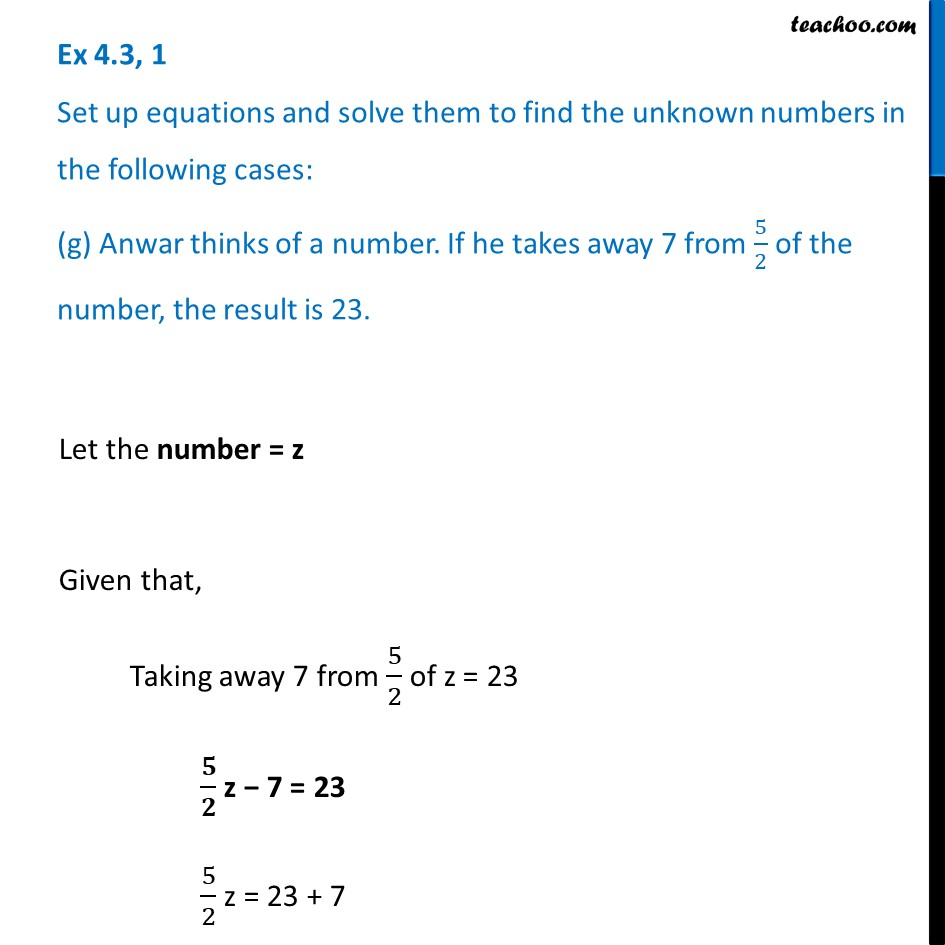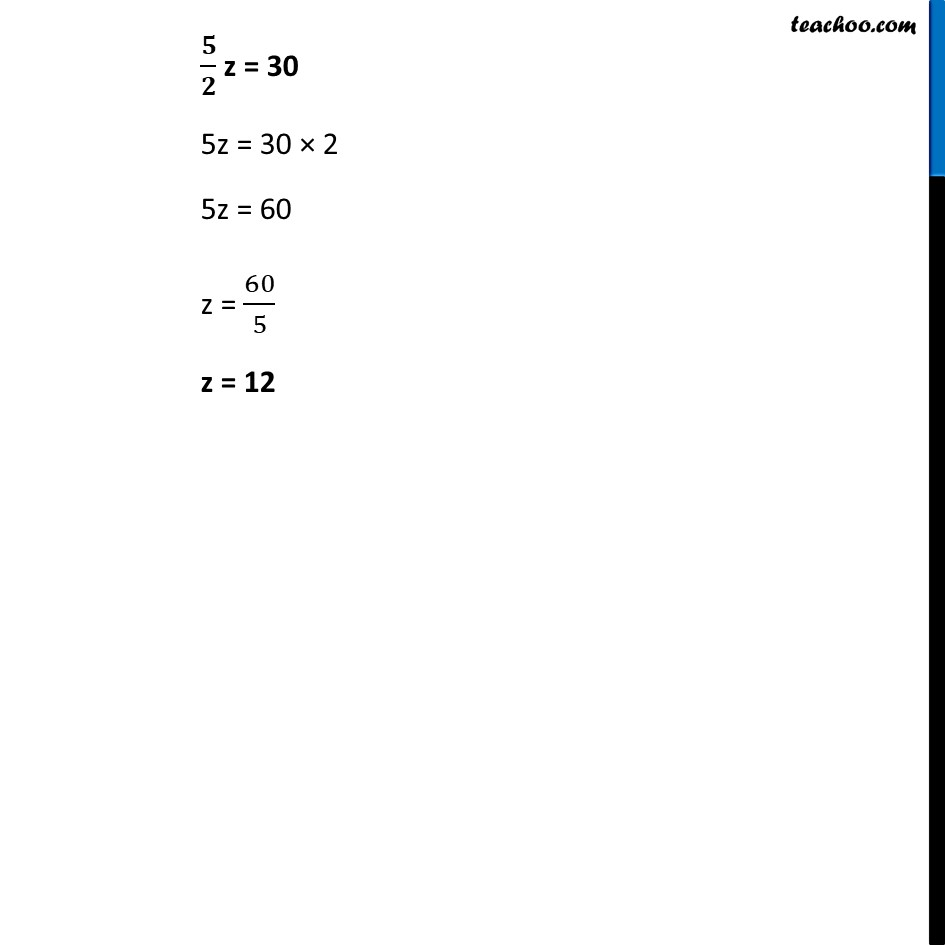Ex 4.3

Chapter 4 Class 7 Simple Equations
Serial order wiseLearn in your speed, with individual attention - Teachoo Maths 1-on-1 Class

### Transcript

Ex 4.3, 1 Set up equations and solve them to find the unknown numbers in the following cases: (g) Anwar thinks of a number. If he takes away 7 from 5/2 of the number, the result is 23.Let the number = z Given that, Taking away 7 from 5/2 of z = 23 𝟓/𝟐 z − 7 = 23 5/2 z = 23 + 7 𝟓/𝟐 z = 30 5z = 30 × 2 5z = 60 z = 60/5 z = 12